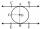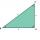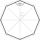# Right triangle - math word problems

1. Tangent 3In a circle with centre O radius is 4√5 cm. EC is the tangent to the circle at point D. Segment AB IS THE DIAMETER of given circle. POINT A is joined with POINT E and POINT B is joined with POINT C. Find DC if BC IS 8cm.
2. Cylinder horizontallyThe cylinder with a diameter of 3 m and a height/length of 15 m is laid horizontally. Water is poured into it, reaching a height of 60 cm below the axis of the cylinder. How many hectoliters of water is in the cylinder?
3. Parallelogram perimeterThe ABC triangle with sides a = 5cm, b = 3cm, c = 40mm has the center of the sides of the K,L,M. How many cm have the KBLM parallelogram perimeter?
4. ChordIt is given to a circle k(r=6 cm) and the points A, B such that / AB / = 8 cm lies on k. Calculate the distance of the center of circle S to the midpoint C of the segment AB.
5. Surface area of the topA cylinder is three times as high as it is wide. The length of the cylinder’s diagonal is 20 cm. Find the surface area of the top of the cylinder.The ladder is 10 m long The ladder is 8 m high How many meters is the distant heel from the wall?What is the slope of a ladder 6.2 m long and 5.12 m in height.
8. Is right triangleDecide if the triangle XYZ is rectangular: x = 4 m, y = 6 m, z = 4 mCalculate the volume and surface area of a regular quadrangular prism 35 cm high and the base diagonal of 22 cm.
10. Right angled triangle 2LMN is a right angled triangle with vertices at L(1,3), M(3,5) and N(6,n). Given angle LMN is 90° find n
11. Body diagonalCuboid with base 7cm x 3,9cm and body diagonal 9cm long. Find the height of the cuboid and the length of the diagonal of the base,
12. The mastA 40 m high mast is secured in half by eight ropes of 25 m long. The ends of the ropes are equidistant from each other. Calculate this distance.
13. Cube wallThe perimeter of one cube wall is 120 meters. Calculate the surface area and the body diagonal of this cube.
14. ABCDAC= 40cm , angle DAB=38 , angle DCB=58 , angle DBC=90 , DB is perpendicular on AC , find BD and AD
15. Perimeter of triangleIn triangle ABC angle A is 60° angle B is 90° side size c is 15 cm. Calculate the triangle circumference.
16. Isosceles triangleThe perimeter of an isosceles triangle is 112 cm. The length of the arm to the length of the base is at ratio 5:6. Find the triangle area.
17. Triangle KLMIn the rectangular triangle KLM, where is hypotenuse m (sketch it!) find the length of the leg k and the height of triangle h if hypotenuse's segments are known mk = 5cm and ml = 15cm
18. Diagonal 20Diagonal pathway for the rectangular town plaza whose length is 20 m longer than the width. if the pathway is 20 m shorter than twice the width. How long should the pathway be?
19. Cube wallCalculate the cube's diagonal diagonal if you know that the surface of one wall is equal to 36 centimeters square. Please also calculate its volume.
20. Rhombus MATHConstruct a rhombus M A T H with diagonal MT=4cm, angle MAT=120°

Do you have an interesting mathematical word problem that you can't solve it? Enter it, and we can try to solve it.

We will send a solution to your e-mail address. Solved examples are also published here. Please enter the e-mail correctly and check whether you don't have a full mailbox.

Please do not submit problems from current active competitions such as Mathematical Olympiad, correspondence seminars etc...